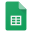# Trendlines

ANALYSIS - Analyze lab data using trendlines.

## Learning Objectives

You should be able to

## What is a Trendline?

A trendline is a line fitted to experimental data that minimizes the square of the error between the values on the line and the values of the data. It is described as a line of best fit. Any trend can be used (e.g. linear, quadratic, logarithmic). See Figure 1 for an example of a linear trendline.Figure 1: An example of a linear trendline.

## Why Does the Technical Audience Value Trendlines?

Trendlines demonstrate a trend in data quickly and visually. If the equation and coefficient of determination (R2) are shown, the results are valuable both qualitatively and quantitatively. The trendline can be used for interpolation of values (estimating values within the tested range) as well as extrapolation of values (estimating values outside the tested range).

## What is a Coefficient of Determination?

The coefficient of determination (aka R2 value) quantifies the ability of the trendline to predict the data. An R2 value of 1.0 indicates that the trendline can be used to predict values exactly, while a value of 0.0 indicates that the trend does not describe the data at all, or conversely that the data do not fit the selected trend. See Figures 2, 3, and 4 for examples of various R2 values.Figure 2. Linear data with linear trendline and perfect correlation.Figure 3. Linear data with significant scatter and poor correlation.Figure 4. Data with no discernable trend and very poor fit.

## What Expectations Does the Technical Audience Have for Plotted Bivariate Data?Figure 5. Linear data with axes labeled and equation and coefficient of determination displayed.

## How Can we Select Appropriate Trends?

Knowing the shape of various functions is critical to selecting an appropriate one for your data. Fortunately, most software packages used for plotting allow you to change your selection easily and compare the coefficient of determination for each. Microsoft Excel provides common trends as options, as seen in Figure 6.

Plotting instruction in Google Sheets is provided here:Figure 6: Trendline options in Microsoft Excel.Trendline Instruction

## References

Vardeman & Jobe (2023) Basic Engineering Data Collection and Analysis (a free open educational resource)